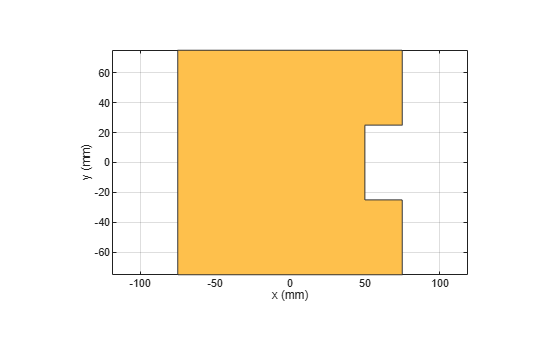# area

Calculate area of 2-D shapes in square meters

## Syntax

``area(shape)``
``a = area(shape)``

## Description

example

````area(shape)` calculates area in sq.m of the specified shape.```

example

````a = area(shape)` calculates and returns area in sq.m of the specified shape.```

## Examples

collapse all

Create a rectangle with a length of 0.15 m, and a width of 0.15 m.

`r = antenna.Rectangle(Length=0.15,Width=0.15);`

Create a second rectangle with a length of 0.05 m, and a width of 0.05 m. Set the center of the second rectangle at half the length of the first rectangle r.

`n = antenna.Rectangle(Center=[0.075,0],Length=0.05,Width=0.05);`

Create and view a notched rectangle by subtracting n from r.

```rn = r-n; show(rn)```Calculate the area of the notched rectangle.

`area(rn)`
```ans = 0.0212 ```

This example shows how to calculate the area of a custom shape created using an rectangle and a circle.

Create a rectangular and a circular shape.

```r = shape.Rectangle; c = shape.Circle;```

Intersect these two shapes to create a custom shape.

```s = r & c; show(s)```Calculate the area in sq.m of the resultant shape.

`area(s)`
```ans = 1.9056 ```

## Input Arguments

collapse all

2-D shape created using shape objects such as:

• `antenna.Rectangle`, `antenna.Circle`, `antenna.Ellipse`, and `antenna.Polygon`, and `antenna.Triangle`.

• `shape.Rectangle`, `shape.Circle`, `shape.Ellipse`, and `shape.Polygon`

Example: ```antenna.Rectangle(Length=2, Width=4)```

Example: `shape.Polygon`

## Output Arguments

collapse all

Area in sq.m of the specified shape returned as a double.

Example: `3.11`

Data Types: `double`

## Version History

Introduced in R2017a